# 置顶如何在小程序中快速实现环形进度条精选热门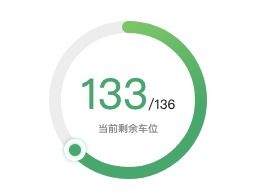[中间的文字部分需要自己实现，因为每个项目不同，本工具只实现进度条]

https://github.com/lucaszhu2zgf/mp-progress

```.canvas{
position: absolute;
top: 0;
left: 0;
width: 400rpx;
height: 400rpx;
}
```

```const context = wx.createContext();
// 打底灰色曲线
context.beginPath();
context.arc(this.convert_length(200), this.convert_length(200), r, 0, 2*Math.PI);
context.setLineWidth(12);
context.setStrokeStyle('#f0f0f0');
context.stroke();

wx.drawCanvas({
canvasId: 'progress',
actions: context.getActions()
});
```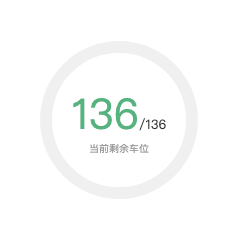```// 圆弧角度
const deg = ((remain/total).toFixed(2))*2*Math.PI;
// 画渐变曲线
context.beginPath();
// 由于外层大小是400，所以圆弧圆心坐标是200,200
context.arc(this.convert_length(200), this.convert_length(200), r, 0, deg);
context.setLineWidth(12);
context.setStrokeStyle('#56B37F');
context.stroke();

// 辅助函数，用于转换小程序中的rpx
convert_length(length) {
return Math.round(wx.getSystemInfoSync().windowWidth * length / 750);
}
```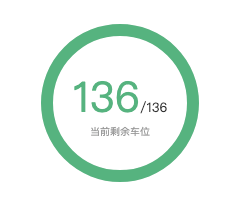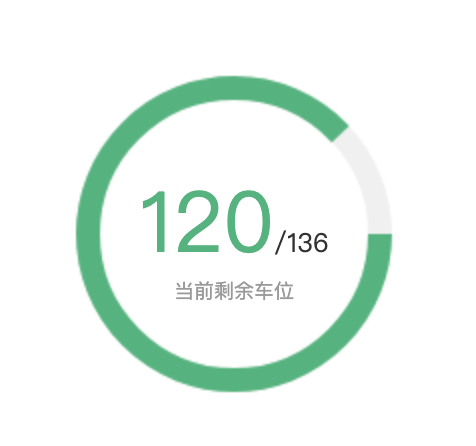```.canvas{
transform: rotate(-90deg);
}
```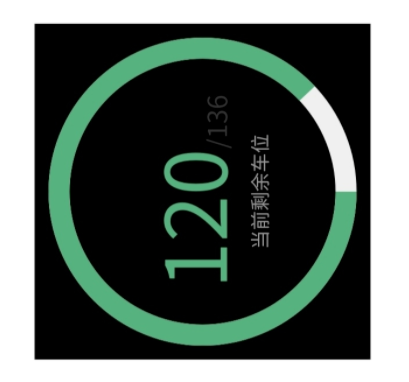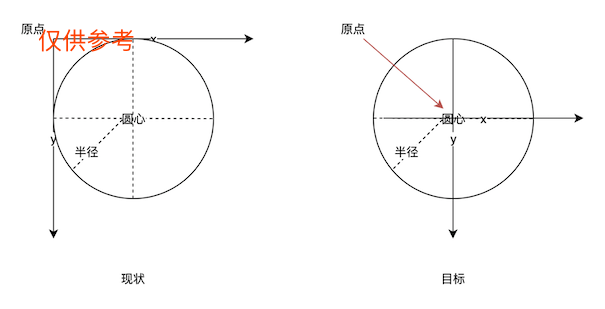```// 更换原点
context.translate(this.convert_length(200), this.convert_length(200));
// arc原点默认为3点钟方向，需要调整到12点
context.rotate(-90 * Math.PI / 180);
// 需要注意的是，原点变换之后圆弧arc原点也变成了0,0
```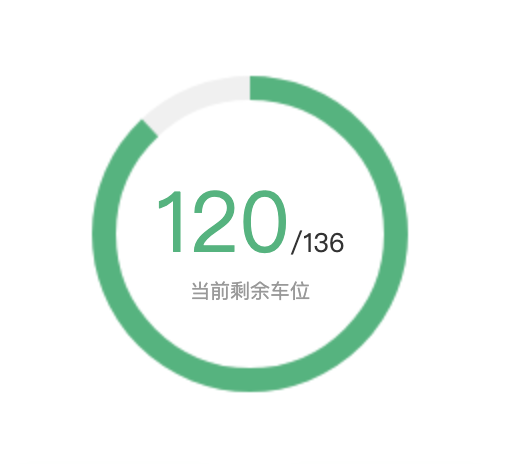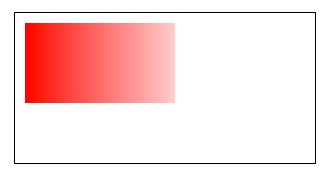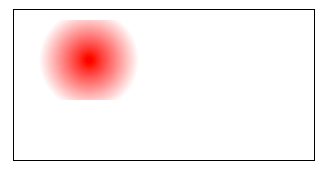```const grd = context.createLinearGradient(0, 0, 100, 90);

// 画渐变曲线
context.beginPath();
context.arc(0, 0, r, 0, deg);
context.setLineWidth(12);
context.setStrokeStyle(grd);
context.stroke();
```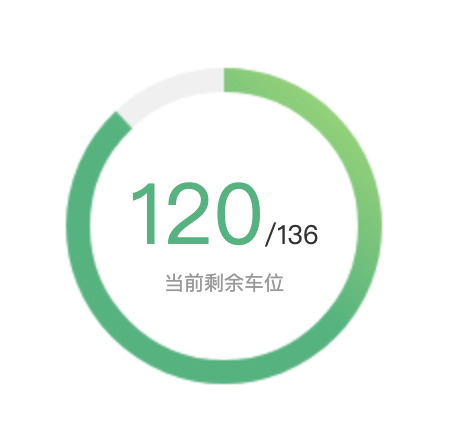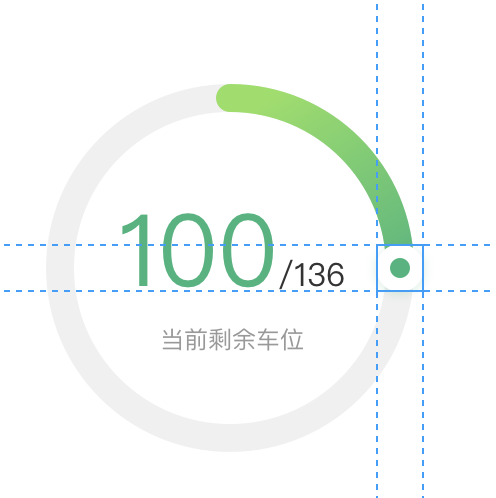```const mathDeg = ((remain/total).toFixed(2))*360;
// 计算弧度
// 圆圈半径
const r = +this.convert_length(170);
// 三角函数cos=y/r，sin=x/r，分别得到小点的x、y坐标
let x = 0;
let y = 0;
if (mathDeg <= 90) {
// 求弧度
} else if (mathDeg > 90 && mathDeg <= 180) {
// 求弧度
} else if (mathDeg > 180 && mathDeg <= 270) {
// 求弧度
} else{
// 求弧度
}
```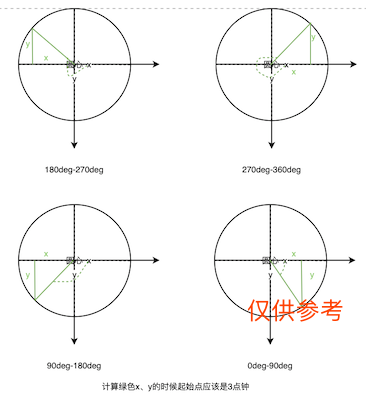```// 画纽扣
context.beginPath();
context.arc(x, y, this.convert_length(24), 0, 2 * Math.PI);
context.setFillStyle('#ffffff');
context.fill();

// 画绿点
context.beginPath();
context.arc(x, y, this.convert_length(12), 0, 2 * Math.PI);
context.setFillStyle('#56B37F');
context.fill();
```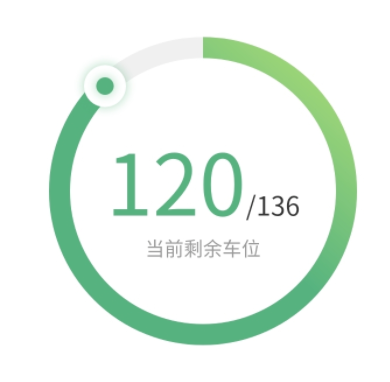20 个评论